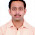Thursday, December 15, 2005

Christos Papadimitriou gave an overview talk at the NIPS conference last week and mentioned the new result by Chen and Deng showing that, given the payoff matrix, the complexity of computing a 2-Player Nash Equilibrium is PPAD-complete.

NIPS is mainly an AI conference and some of my Machine Learning friends, who have a good understanding of NP-completeness, went around asking who knew what PPAD-complete meant. They got many blank stares and a few confusing answers.

So what is PPAD? Let's start with TFNP (Total Functions in NP). Consider a polynomial-time computable predicate P(x,y) where for every x there is at least one y such that P(x,y) is true. A TFNP function is the problem of given an input x, finding a y such that P(x,y).

The interesting TFNP functions come from combinatorial theorems that guarantee solutions. One such theorem, called the Polynomial Parity Argument (PPA), is given a finite graph consisting of lines and cycles (every node has degree at most 2), there is an even number of endpoints.

The class PPAD is defined using directed graphs based on PPA. Formally PPAD is defined by its complete problem (from an earlier post): Given an exponential-size directed graph with every node having in-degree and out-degree at most one described by a polynomial-time computable function f(v) that outputs the predecessor and successor of v, and a vertex s with a successor but no predecessors, find a t≠s that either has no successors or no predecessors.

Besides two player Nash, arbitrary k-player Nash and a discrete version of finding Brouwer fixed points are also complete for PPAD.

So how do we explain this Nash Equilibrium result say when we try to explain the result to economists and AI folk. Just say Nash Equilibrium for 2-player games has the same complexity as k-player Nash and finding fixed points and we have some evidence that no efficient solutions exist for such problems.

1.Any pointers to the evidence of intractibility?

2.I point to the lack of pointers to evidence of tractibility--that's usually how things go in this field.

3.Also, if you count oracle results as evidence: PPAD is different from FP relative to a generic oracle, if I recall a result of Beame, Cook, Edmonds, Impagliazzo and Pitassi correctly.

4.While oracle results are very useful in understanding the landscape of complexity theory, I think they are somewhat less useful in "making a case" that some problem is hard, especially to non-theoreticians.

5.Wouldn't the existence of one-way permutations (perhaps with some technical conditions, like efficient verifiability that a value lies in the correct range) imply that PPAD is hard?

6.is TFNP subset of NP?

1.